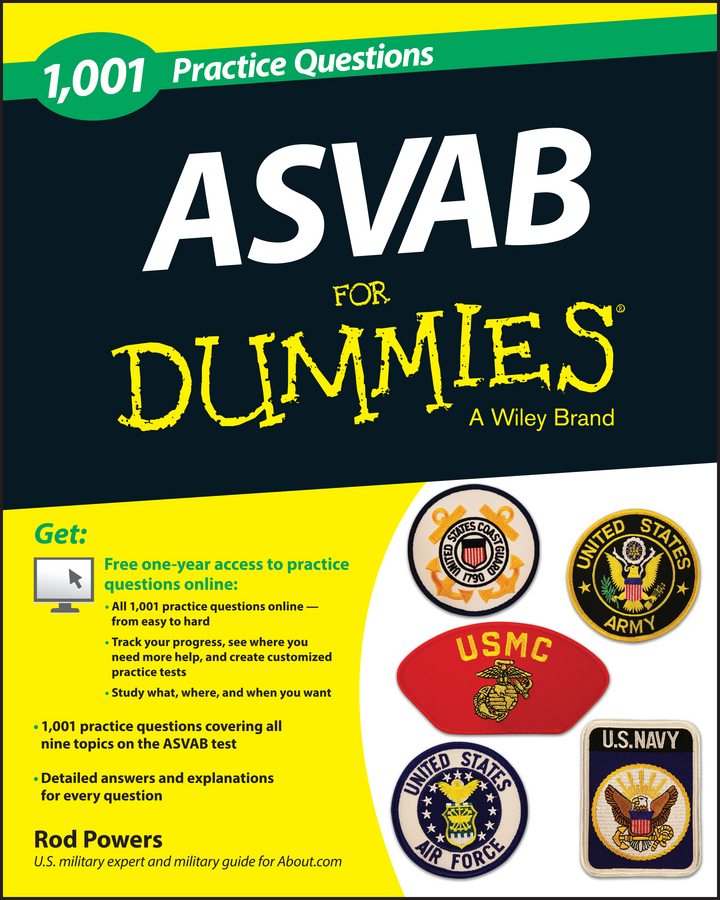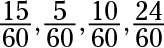##### 1,001 ASVAB Practice Questions For Dummies (+ Free Online Practice)Use these ASVAB questions to get used to the general test structure and to practice some of the concepts you need to know for this test. When you’re ready, take a full blown practice exam.

## Sample questions

1. If apples are on sale at 15 for \$3, what is the cost of each apple?

• (A)50¢

• (B)25¢

• (C)20¢

• (D)30¢

2. A noncommissioned officer challenged her platoon of 11 enlisted women to beat her record of performing a 26-mile training run in four hours. If all the enlisted women match her record, how many miles will they have run?

• (A)71.5 miles

• (B)6.5 miles

• (C)286 miles

• (D)312 miles

3. Margaret gets her hair cut and colored at an expensive salon in town. She is expected to leave a 15 percent tip for services. If a haircut is \$45 and a color treatment is \$150, how much of a tip should Margaret leave?

• (A)\$22.50

• (B)\$29.25

• (C)\$20.00

• (D)\$195.00

4. A bag of sand holds 1 cubic foot of sand. How many bags of sand are needed to fill a square sandbox measuring 5 feet long and 1 foot high?

• (A)5 bags

• (B)10 bags

• (C)15 bags

• (D)25 bags

5. The day Samantha arrived at boot camp, the temperature reached a high of 90 degrees in the shade and a low of –20 degrees at night in the barracks. What is the average between the high and low temperatures for the day?

• (A)35 degrees

• (B)45 degrees

• (C)70 degrees

• (D)62 degrees

6. Farmer Beth has received an offer to sell her 320-acre farm for \$3,000 per acre. She agrees to give the buyer \$96,000 worth of land. What fraction of Farmer Beth’s land is the buyer getting?

• (A)1/4

• (B)1/10

• (C)1/5

• (D)2/3

7. A map is drawn so that 1 inch equals 3 miles. On the map, the distance from Kansas City to Denver is 192.5 inches. How far is the round trip from Kansas City to Denver in miles?

• (A)192.5 miles

• (B)577.5 miles

• (C)385 miles

• (D)1,155 miles

8. Margaret and Julie can sell their store for \$150,000. They plan to divide the proceeds according to the ratio of the money they each invested in the business. Margaret put in the most money, at a 3:2 ratio to Julie. How much money should Julie get from the sale?

• (A)\$50,000

• (B)\$30,000

• (C)\$60,000

• (D)\$90,000

9. In the military, 1/4 of an enlisted person’s time is spent sleeping and eating, 1/12 is spent standing at attention, 1/6 is spent staying fit, and 2/5 is spent working. The rest of the time is spent at the enlisted person’s own discretion. How many hours per day does this discretionary time amount to?

• (A)6 hours

• (B)1.6 hours

• (C)2.4 hours

• (D)3.2 hours

10. Train A is headed east at 55 mph. Train B is also heading east on an adjacent track at 70 mph. At the end of four hours, how much farther will train B have traveled than train A?

• (A)40 miles

• (B)50 miles

• (C)60 miles

• (D)70 miles

Use this answer key to score the practice Arithmetic Reasoning questions.

1. C. Divide \$3 by 15.

2. C. Multiply 26 × 11. The other information in the question is irrelevant; it’s there to throw you off.

3. B. Add \$45 and \$150 and multiply the answer by 15 percent, or 0.15.

4. D. The volume formula for a square or rectangular box is v = lwh, so v = 5 × 5 × 1 = 25 cubic feet. Each bag holds 1 cubic foot of sand.

5. A. Add the two temperatures given and then divide by the number of terms, 2: (90 + –20) ÷ 2 = 70 ÷ 2 = 35.

6. B. \$96,000 divided by \$3,000 (the price per acre) equals 32 acres, and 32 acres divided by 320 acres (the total size of the farm) equals 10 percent, or 1/10 of the land.

7. D. Multiply 192.5 × 3 to get the distance in miles and then double the answer to account for both legs of the trip.

8. C. Margaret should get 3/5 of the money and Julie should get 2/5 of the money. The fractions are calculated by adding both sides of the ratio together (3 + 2 = 5) to determine the denominator. Each side of the ratio then becomes a numerator, so Margaret’s investment can be shown to be 3/5 of the total investment, and Julie’s is 2/5 of the total investment.

You can check these fractions by adding 3/5 and 2/5 to get 5/5 or 1, which is all the money.) Divide \$150,000 by 5, and then multiply the answer by 2 to determine Julie’s share of the money.

9. C. Calculate this answer by first assigning a common denominator of 60 to all the fractions and adjusting the numerators accordingly:Add the fractions to find out how much time is allotted to all these tasks. The total is 54/60, which leaves 6/60 or 1/10 of the day to the enlisted person’s discretion. 1/10of 24 hours is 2.4 hours.

10. C. The distance formula is d = rt. Plug in the known values:

• Train A: d = 55 × 4 = 220 miles

• Train B: d = 70 × 4 = 280 miles

Train B traveled 280 – 220 = 60 miles farther than train A.